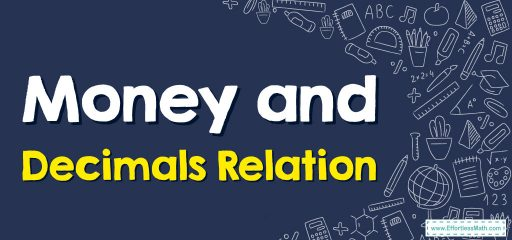# Money and Decimals Relation: A Step-by-Step Guide

You can relate money to decimals in several ways. The money or financial system is centered on a decimal system of ones, tens, hundreds, etc. on the whole number or the left-hand side of the decimal point and tenths and hundredths are on the right-hand or decimal side.## A step-by-step guide to relating money and decimals.

Here is a step-by-step guide to understanding the relationship between money and decimals.

### Step 1: Understand Decimal Basics

Decimals are a way of representing fractions where the denominator is a power of ten. The decimal point separates the whole number part from the fractional part of a number. For example, in the number 1.25, 1 is the whole number part, and 0.25 is the fractional part.

### Step 2: Understand the Place Value in Decimals

The first digit after the decimal point is the tenths place. This represents 1/10th. The second digit after the decimal point is the hundredths place, representing 1/100th. So, in 1.25, 2 is in the tenths place and 5 is in the hundredths place.

### Step 3: Relate Decimals to Money

Now, let’s relate this to money. In most currencies, like dollars or euros, 1 unit of currency can be broken down into 100 smaller units, like cents or pennies. This maps perfectly onto the decimal system, where 1.00 can be broken down into .01 units.

### Step 5: Adding and Subtracting Money

When adding or subtracting money, it’s just like adding or subtracting decimals. Just line up the decimal points and perform the operation. For example, if you have $2.75 and you spend$1.50, you would subtract to find out how much money you have left:

1.50

So, you would have $1.25 left. ### Step 6: Multiplying and Dividing Money When multiplying or dividing money amounts, treat it just like regular decimal multiplication or division. However, remember that the result may not always be a practical amount of money. For example, if you divide$5.00 by 2, you would get $2.50. But if you divide$5.00 by 3, you would get approximately $1.67, not an exact amount in cents. Remember to practice these steps, as understanding money and decimals is a fundamental skill in math and everyday life. ### Example 1: What are the missing numbers to complete the addition sentences?$6.32 = … dollars  … dimes  … pennies.

$6.32 = … ones … tens … hundreds. Solution:$6.32 is the same as 6 dollars and 32 cents. So, $6.32 = 6 dollars + 3 dimes + 2 pennies. To find out how decimals relate to money, think of dollars as ones, dimes as tenths, and pennies as hundredths. So,$6.32 = 6 ones + 3 tenths + 2 hundredths.

### What people say about "Money and Decimals Relation: A Step-by-Step Guide - Effortless Math: We Help Students Learn to LOVE Mathematics"?

No one replied yet.

X
30% OFF

Limited time only!

Save Over 30%

SAVE $5 It was$16.99 now it is \$11.99